## THE WOOLY WORM RACE [DAY 2]

### FRACTIONS & METRIC UNITS

Represent, compare, order, and add fractions involving metric unit conversions.

## Intentionality & Unit Overview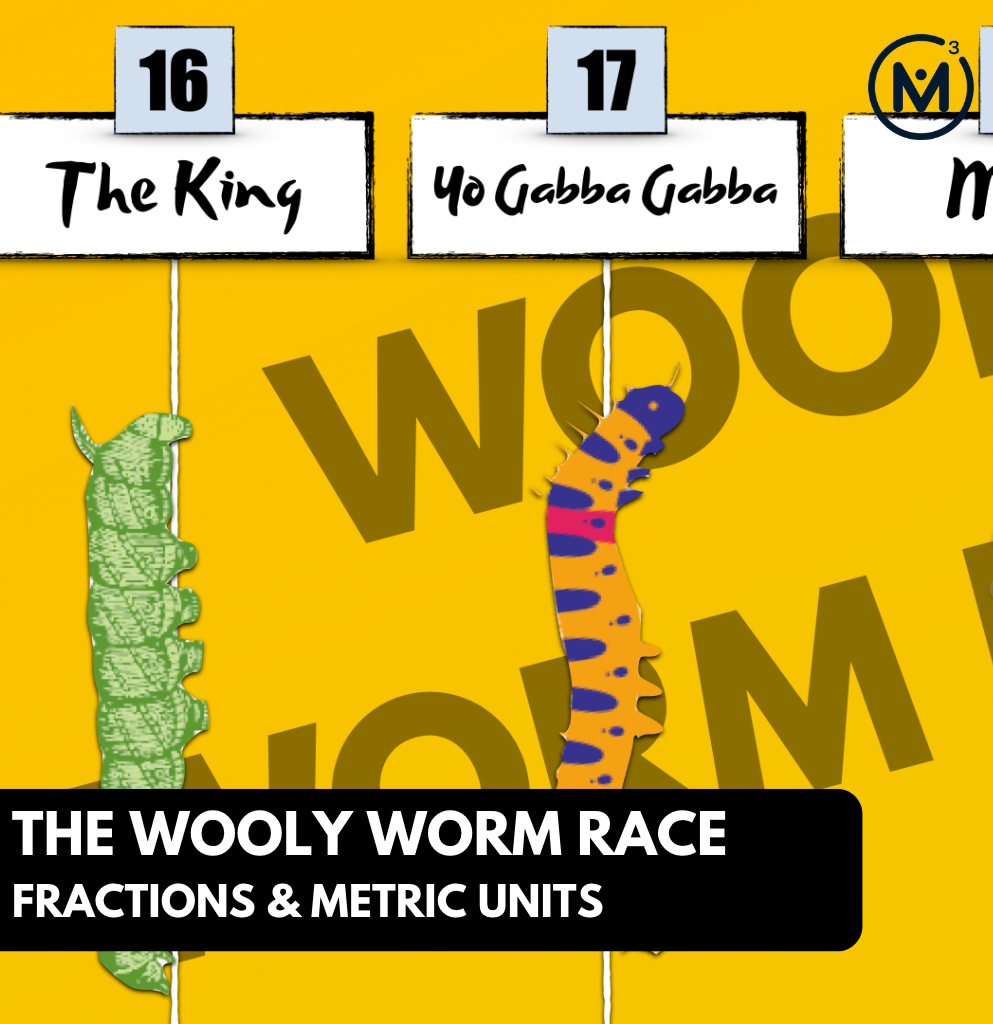### Access each lesson from this unit using the navigation links below

Students will explore representing, comparing and ordering fractions. They will also have an opportunity to determine appropriate units of measure and the relationship between larger and smaller units.

## Intentionality…

The purpose of the Day 2 activities is to reinforce key concepts from Day 1. Students will engage in a string of related problems through a math talk and will have an opportunity to complete independent purposeful practice. The math talk and purposeful practice serve to develop a deeper understanding of the following big ideas.

Number:

• Fractions can be represented in a variety of ways;
• How you partition the whole determines the fractional unit (i.e.: partitioning a whole into 6 parts will result in 6 sixth parts);
• As you partition a whole into more parts, the smaller the size of each part;
• As you partition a whole into more parts, the larger the denominator;
• In order to compare two or more fractional quantities, the whole must be the same;
• The numerator indicates the number of parts relative to the number of parts in the whole indicated by the denominator; and,
• Fractional amounts exist between whole numbers.

Measurement:

• Units of measure have different sizes and attributes; and,
• As the size of the unit of measure decreases, the number of iterations of that unit required to measure a quantity increases (and vice versa).

## Math Talk #1

In today’s visual math talk, students will be asked to represent different fractional amounts on a number line from 0 to 2. The goal of this visual math talk is to build student flexibility and fluency with fractional quantities while also practicing partitioning a linear model.

The prompt for each problem in this visual math talk is:

Where would you place each fractional amount on the number line?

However, consider leveraging yesterday’s Wooly Worm Race to frame our 0 to 2 number line to represent the 2 metre track that the caterpillars would race along.

Introduce each quantity, one at a time, for students to place on a number line.

One

One-half

1/4

One-eighth

6/8

Login/Join to view three (3) additional Visual Math Talk Prompts as well as the Teacher Guide, downloadable slide decks and printable handouts for this lesson and all problem based units.

In today’s math talk, we intentionally switch between words and standard notation. We want students to begin connecting the two symbolic representations of a fraction and to continue developing their ability to spatially partition a linear model.

Encourage students to draw a new number line from 0 to 2 for each prompt in order to promote additional practice of partitioning as well as to ensure they do not get confused with partitions that were helpful for one prompt, but not so helpful for another.

If necessary, consider providing students with strips of paper to practice paper folding as a tool for this math talk.

Although you could lead this math talk by orally sharing the context and representing student thinking on a chalkboard/whiteboard, consider leveraging the following visual math talk prompt videos to ensure accessibility for all students. We would recommend that you still model student thinking to ensure student voice is honoured prior to sharing the visual silent solution shared in the visual prompt video.

## Visual Math Talk Prompt #1

Begin playing the first video to share the first visual math talk prompt and be ready to pause the video in order to give students time to think.

The prompts are simple and shared without context, however since this is taken from Day 2 of the Wooly Worm Race problem based math unit, you might consider leveraging the context from the previous day involving caterpillars racing along a 2 metre straight track. In that case, you could verbally share the context using a script such as:

The Wooly Worms are racing in their next competition!

Woolzy managed to race 1 metre.

Where on the track from 0 metres to 2 metres did Woolzy finish?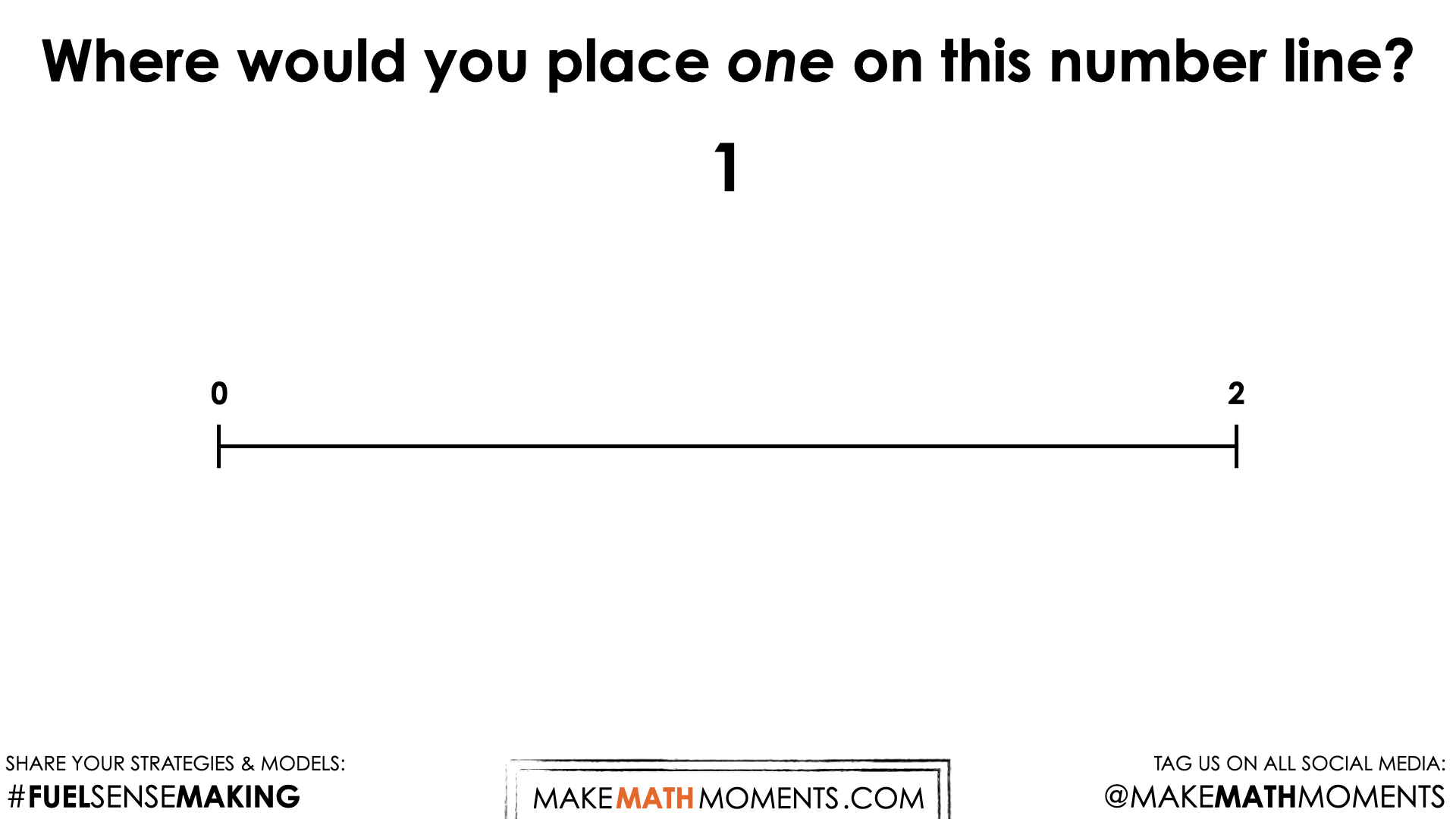In this first prompt, students will likely have no problem partitioning the 0 to 2 number line into two parts and indicating a value of 1 at the halfway point.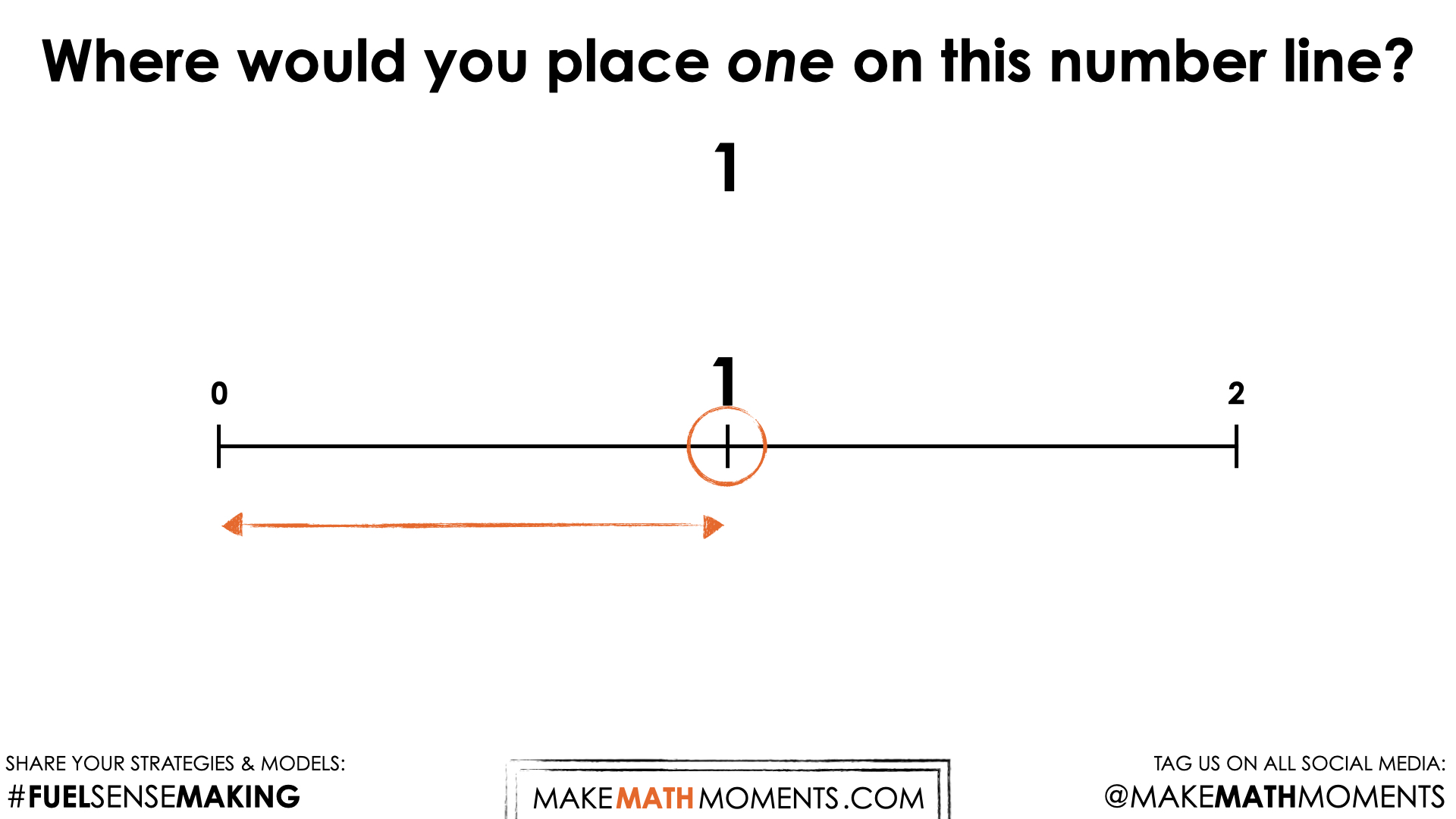## Visual Math Talk Prompt #2

Playing the second video until prompted to pause and consider leveraging the Wooly Worm context describing where the second caterpillar finished the race at the 1 half metre mark.

Encouraging students to draw another number line rather than simply building on the first number line will give them the opportunity to (likely) partition the number line in half and then partition each half in half.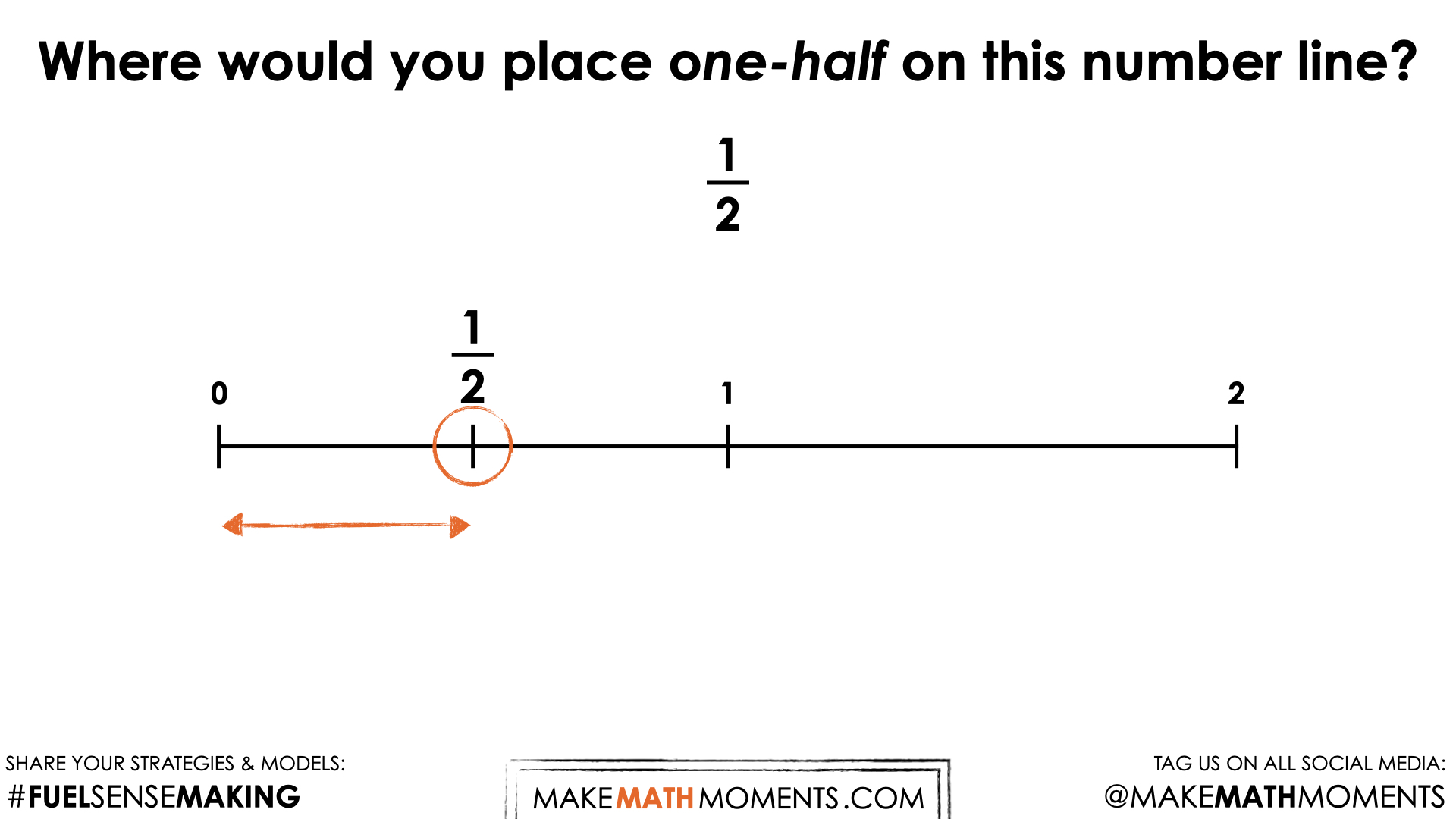Depending on the readiness of the learners, you might consider mentioning that we have essentially taken one half of one half which can be written symbolically as ½ x ½ to emerge the idea of multiplying fractions.

## Visual Math Talk Prompt #3

Play the third video until prompted to pause and consider leveraging the same context verbalizing something similar to:

Show where the third caterpillar finished the race at the ¼ metre mark on the 2 metre long track.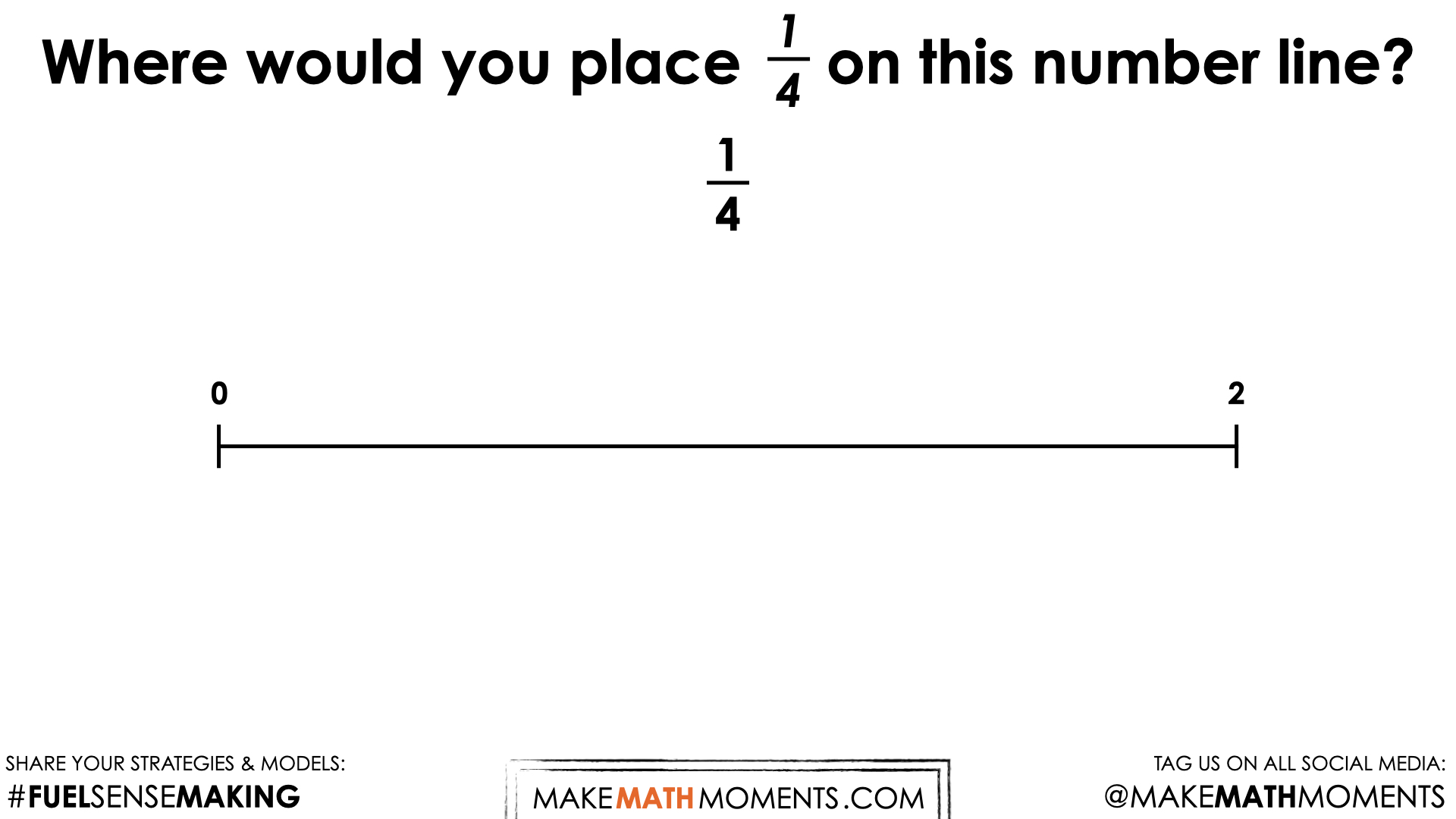Again, it is nice to encourage students to draw another number line for this prompt, however they can ultimately decide how they choose to model their thinking.

In this case, students are halving the 0-2 number line, then halving those resulting parts and then finally, halving those parts again to reveal ¼ on the 0-2 number line.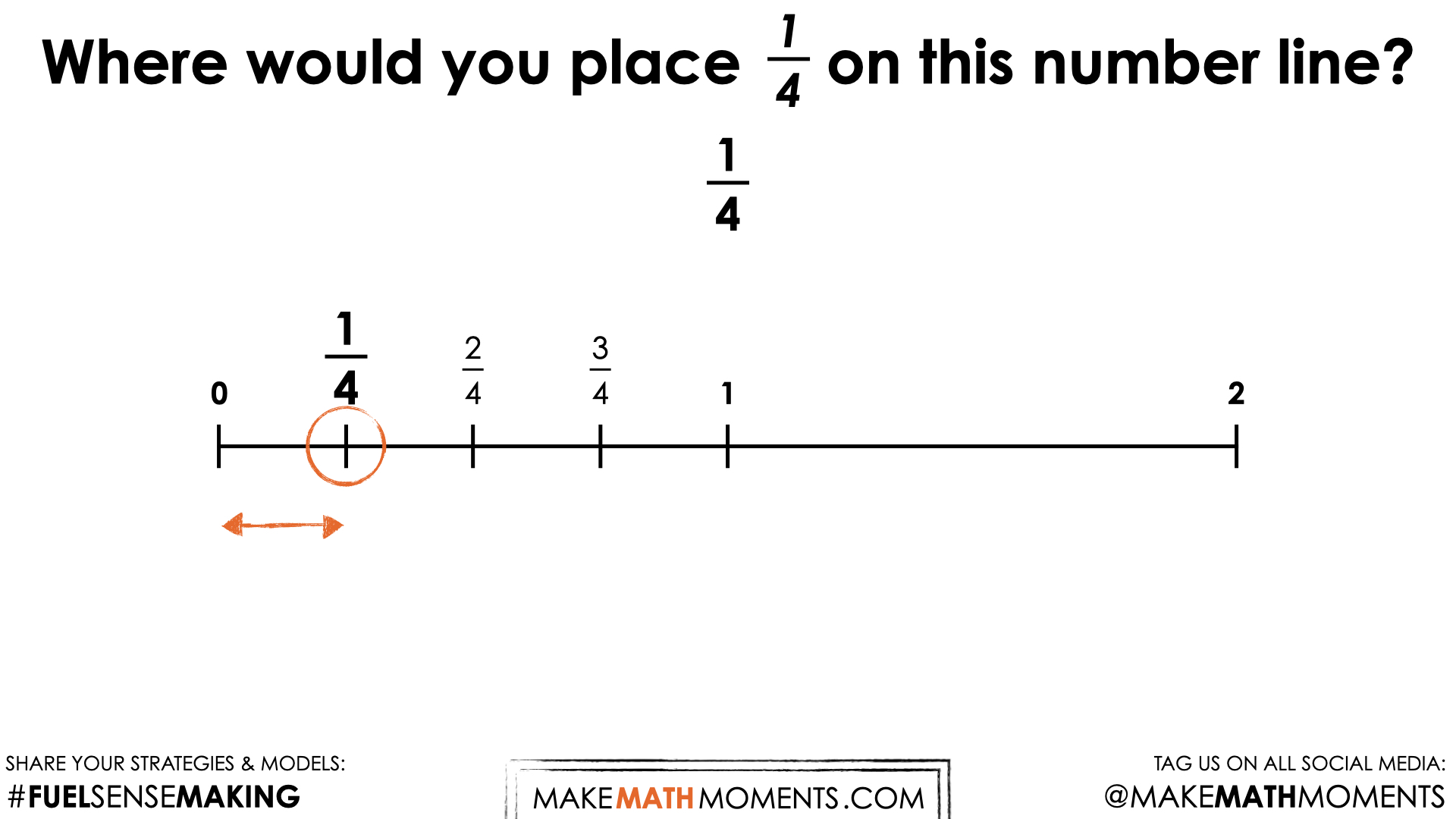There are a number of different ways we could represent this symbolically. For example, we could say that we took half of a half of a half of the 0-2 number line:

½ of ½ of ½ of 2

= ½ x ½ x ½ x 2

= ½ x ½ x (½ x 2)

= ½ x ½ x (1)

= ½ x (½ x 1)

= ½ x (½)

= ½ x ½

= ¼

Is this symbolic representation a bit of overkill? Absolutely. However, if this helps students better connect multiplying fractions symbolically to the act of partitioning our number line repeatedly, then it could be worth the while.

## Visual Math Talk Prompt #4

Playing the following video until prompted to pause and consider leveraging the same context verbalizing something similar to:

Show where the third caterpillar finished the race at the ⅛  metre mark on the 2 metre long track.

Again, we are going to be encouraging students to use a new 0 to 2 number line and begin the process of partitioning to determine where 1 eighth would be placed on the number line.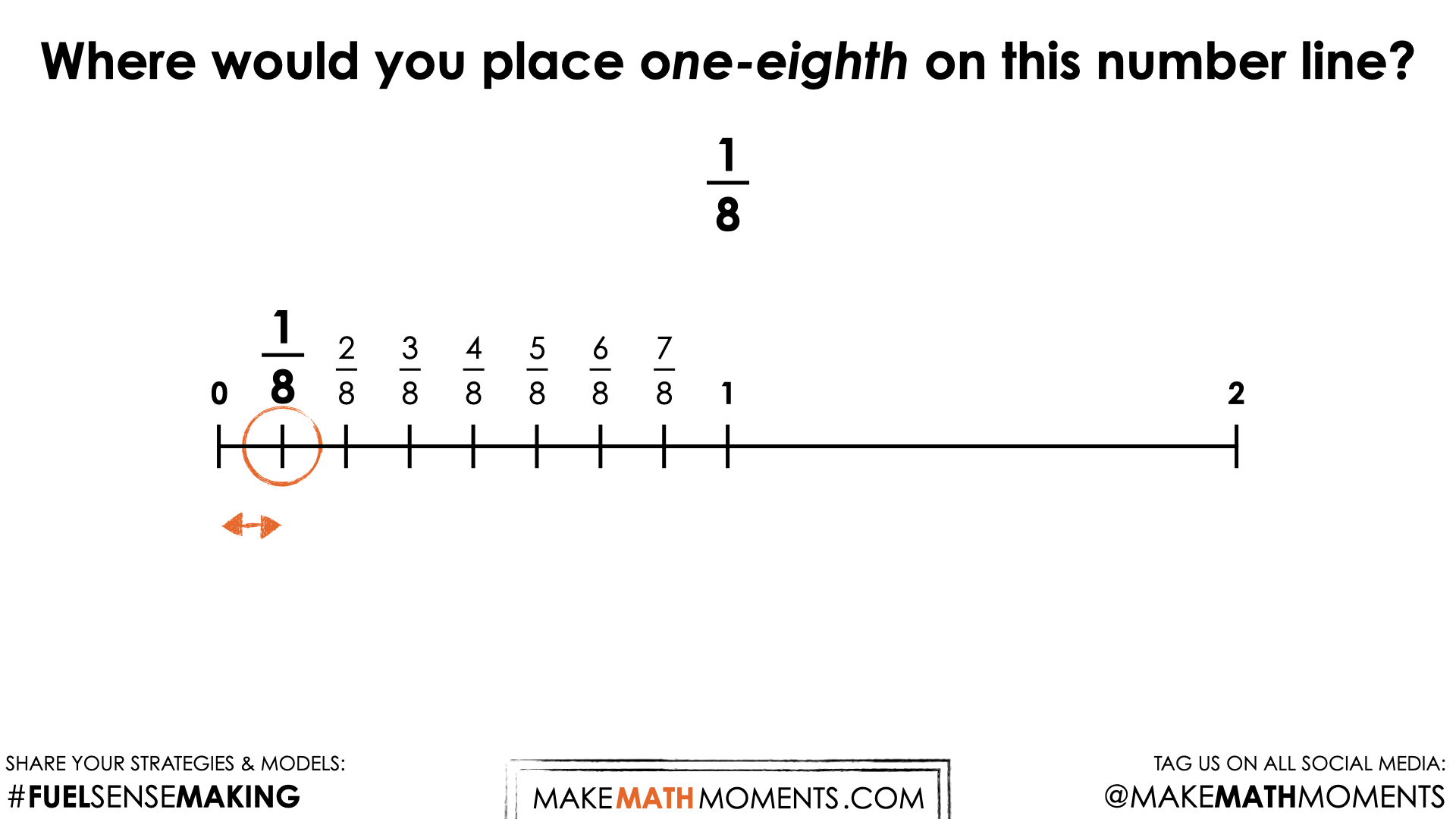Consider asking students to elaborate on what they have ultimately done to determine 1 eighth of a metre.

## Visual Math Talk Prompt #5

Play the video until prompted to pause and consider leveraging the same context verbalizing something similar to:

Show where the third caterpillar finished the race at the 6/8 metre mark on the 2 metre long track.

With this particular prompt, you might not require students to re-partition a brand new number line, but rather to articulate where 6 eighths would be placed on the number line.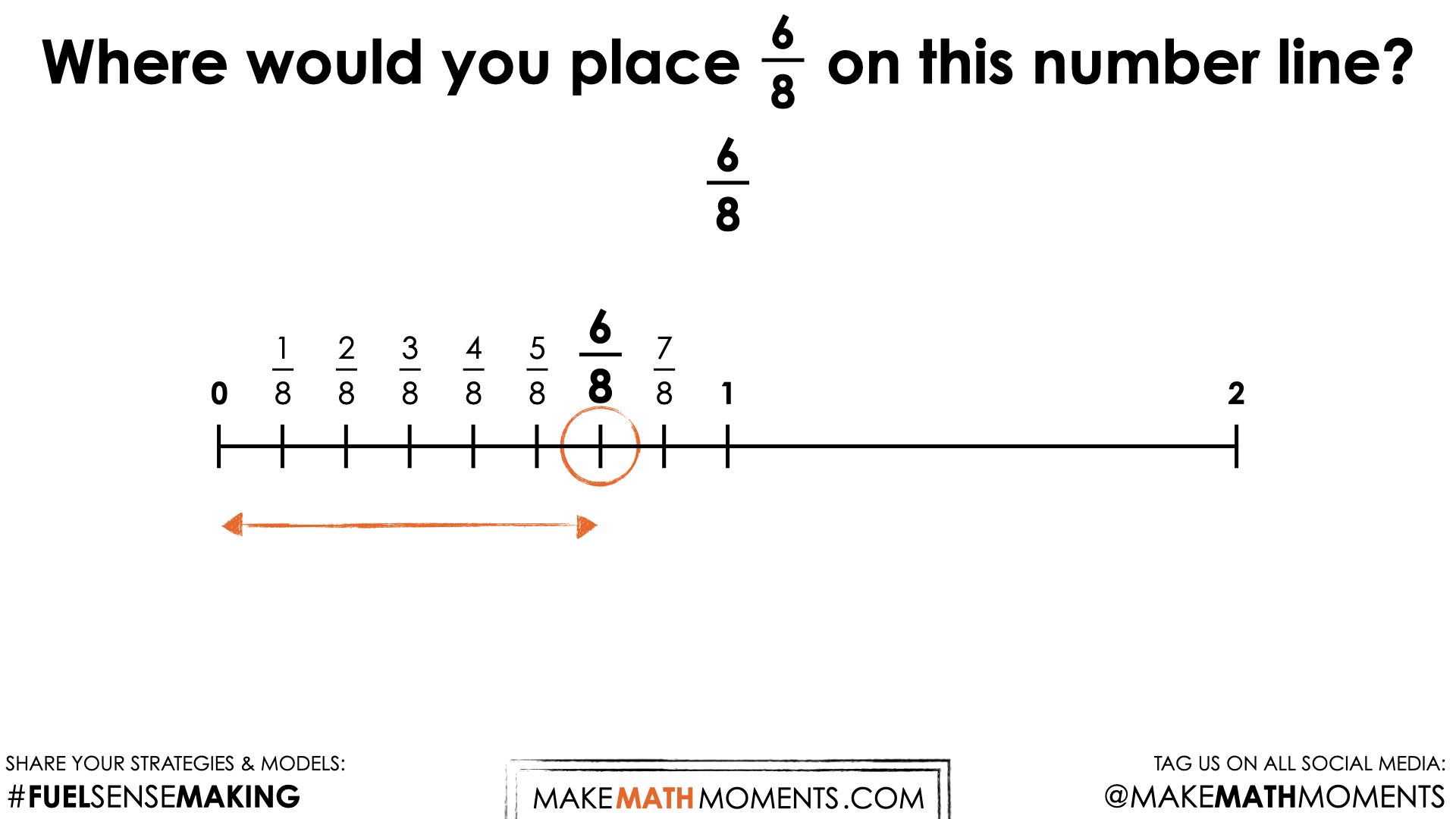Consider asking students to elaborate on what they have ultimately done to determine how they landed on 6 eighths of a metre.

For example, some students might argue that they found 6 groups of half of a half of a half of a half of 2 metres which could be written symbolically as:

6 x (½ of ½ of ½ of ½ of 2)

Are there any other ways that we could represent what was done to partition the line and determine where 6 eighths of a metre would be?

## Visual Math Talk Prompt #6

Login/Join to view three (3) additional Visual Math Talk Prompts as well as the Teacher Guide, downloadable slide decks and printable handouts for this lesson and all problem based units.

## Visual Math Talk Prompt #7

Login/Join to view three (3) additional Visual Math Talk Prompts as well as the Teacher Guide, downloadable slide decks and printable handouts for this lesson and all problem based units.

## Visual Math Talk Prompt #8

Login/Join to view three (3) additional Visual Math Talk Prompts as well as the Teacher Guide, downloadable slide decks and printable handouts for this lesson and all problem based units.

## Math Talk #2

Login/Join to view this additional set of Math Talk Prompts as well as the Teacher Guide, downloadable slide decks and printable handouts for this lesson and all problem based units.

## While Students Are Practicing…

Login/Join to access the entire Teacher Guide, downloadable slide decks and printable handouts for this lesson and all problem based units.

## Questions: Working With Fractions & Metric Units

Login/Join to access the entire Teacher Guide, downloadable slide decks and printable handouts for this lesson and all problem based units.

Login/Join to access the entire Teacher Guide, downloadable slide decks and printable handouts for this lesson and all problem based units.

## Educator Discussion Area

Login/Join to access the entire Teacher Guide, downloadable slide decks and printable handouts for this lesson and all problem based units.

## Explore Our 60+ Problem Based Units

This Make Math Moments Lesson was designed to spark curiosity for a multi-day unit of study with built in purposeful practice, number talks and extensions to elicit and emerge strategies and mathematical models.

Dig into our other units of study and view by concept continuum, grade or topic!